### Home > PC > Chapter 6 > Lesson 6.4.2 > Problem6-161

6-161.
1. Suppose y varies inversely as x + 6 and y = 1 when x = 1. Homework Help ✎

1. Express y as a function of x, y = f(x).

2. Find f(−3), f(0), f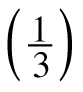, and f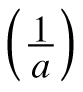.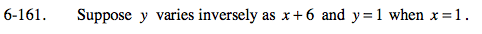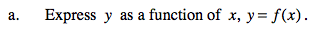1. Write the inverse proportion. Don't forget 'k'.
2. Substitue a point and solve for 'k'.
3. Write the function.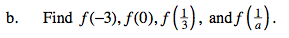Use the function found in part (a) above.